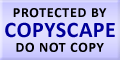Submit Assignment From Here
Name
:
Email
:
Phone
:
Country
:
Willing to pay US\$
:
Subject
:
Topic
:
Levels
:
:Hours
:

# Two-Sample Hypothesis Tests Homework Help

Two-Sample Hypothesis Tests is a statistical analysis tool which helps in detecting if there is any difference between the means of two different populations. In Two-Sample Hypothesis Tests we compare two different parameters from two different populations. The example of Two-Sample Hypothesis Tests is the difference in the mean income of the male and female doctors in the country.
For a Two-Sample Hypothesis Tests we consider a null hypothesis and an alternative hypothesis. The null hypothesis states that there is no difference between the parameters of the two different populations which are being considered. It always contains greater than equal to, equal to or less than equal to signs. On the other hand alternate hypothesis is proved when null hypothesis is considered to be false. The symbols contained in alternate hypothesis are greater than, not equal to, and less than signs.
Three conditions are required to be satisfied to evaluate the difference between the mean of two populations. These conditions are as followed:
1) The selection of sample must be randomly based.
2) The sample has to be independent. Independence can be verified by the relationship among the samples. If the samples are not related to each other they are said to be independent.
3) The minimum size of the sample should be 30; if the sample size is not present then the population must have standard deviation.

 Click here to submit assignment Statistics Homework Help Confidence Interval Estimation Continuous Probability Distribution Methods of Least Squares and Curve Fitting Estimation Theory Numerical Measures Principles of Data Reduction Properties of Random Sample

### Live Online Tutoring### Make Payment OnlineUS